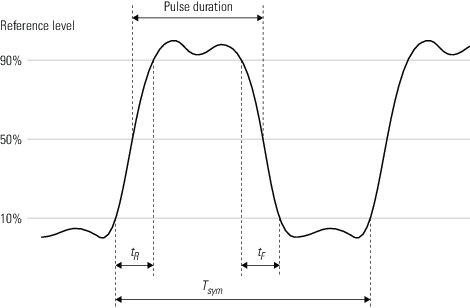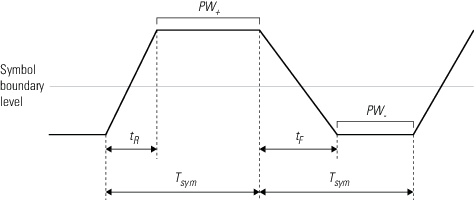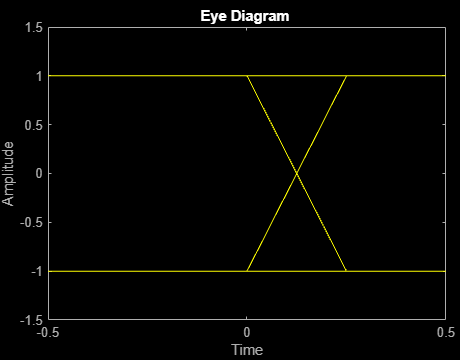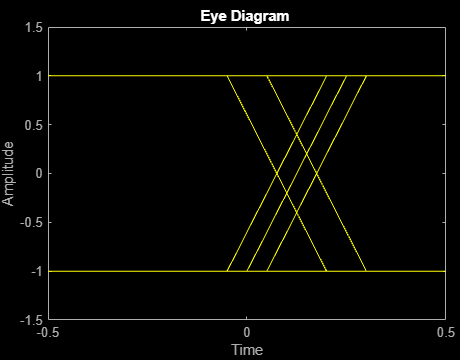# commsrc.pattern

Construct pattern generator object

## Syntax

``h = commsrc.pattern``
``h = commsrc.pattern(Name,Value)``

## Description

example

````h = commsrc.pattern` constructs a pattern generator object, `h`.The pattern generator object produces modulated data patterns. The object can be used to inject jitter into modulated signals.```
````h = commsrc.pattern(Name,Value)` creates a combined jitter generator object with the specified property `Name` set to the specified `Value`. You can specify additional name-value pair arguments in any order as (`Name1`,`Value1`,...,`NameN`,`ValueN`).```

## Properties

A pattern generator object includes these properties. You can edit all properties, except those explicitly noted.

PropertyDescription
`Type`Type of pattern generator object (`'Pattern Generator'`). This property is not writable.
`SamplingFrequency`

Sampling frequency of the input signal in hertz.

`SymbolRate`The symbol rate of the input signal. This property depends upon the `SamplingFequency` and `SamplesPerSymbol` properties. This property is not writable.
`SamplesPerSymbol`

The number of samples representing a symbol. `SamplesPerSymbol` must be an integer. This property affects `SymbolRate`.

`PulseType`

The type of pulse the object generates. Pulse types available: return-to-zero (`'RZ'`) and non-return-to-zero (`'NRZ'`). The initial condition for an `'NRZ'` pulse is `0`.

`OutputLevels`

Amplitude levels that correspond to the symbol indices. For an `'NRZ'` pulse, specify as a 1-by-2 vector. The first element of the 1-by-2 vector corresponds to the 0th symbol (data bit value 0). The second element corresponds to the 1st symbol (data bit value 1). For an `'RZ'` pulse, specify as a scalar and the value corresponds to the data bit value `1`.

`DutyCycle`

The duty cycle of the pulse the object generates. Displays calculated duty cycle based on pulse parameters. This property is not writable.

`RiseTime`

Specifies 10% to 90% rise time of the pulse in seconds.

`PulseDuration`

Pulse duration in seconds defined by IEEE STD 181 standard. See the Return-to-Zero (RZ) Signal Conversion: Ideal Pulse to STD–181 figure in the Object Functions. Applies when `PulseType` is `'RZ'`.

`FallTime`

Fall time of the pulse in seconds, specified as a percentage from 10 to 90.

`DataPattern`

The bit sequence the object uses, specified as `'PRBS5'`, `'PRBS6'`, …, `'PRBS15'`, `'PRBS23'`, `'PRBS31'`, and`'User Defined'`.

`UserDataPattern`

User-defined bit pattern consisting of a vector of ones and zeroes. Applies when `DataPattern` is `'User Defined'`.

`Jitter`

Jitter characteristics, specified as a `commsrc.combinedjitter` object. Use this property to configure Random, Periodic and Dual Dirac Jitter.

## Object Functions

A pattern generator object has five object functions, as described in this section.

### generate

This object function outputs a frame worth of modulated and interpolated symbols. It has one input argument, which is the number of symbols in a frame. Its output is a column vector. You can call the object function using this syntax:

`x = generate(h, N)`
where `h` is the handle to the object, `N` is the number of output symbols, and `x` is a column vector whose length is `N` multiplied by `h.SamplesPerSymbol`.

### reset

This object function resets the pattern generator to its default state. The property values do not reset unless they relate to the state of the object. This object function has no input arguments.

### idealtostd181

This object function converts the ideal pulse specifications to IEEE STD-181 specifications. The ideal 0% to 100% span rise time (`tr`) and fall time (`tf`) are converted to 10% to 90% spans with a 50% pulse width duration (`pw`). Call the `idealtostd181` object function using this syntax:

`h = idealtostd181(tr,tf,pw)`

The object function sets the appropriate properties. The IEEE STD-181 Return-to-Zero (RZ) signal parameters are shown in this figure.### std181toideal

The `std181toideal` object function converts the IEEE STD-181 pulse specifications, stored in the pattern generator, to ideal pulse specifications. The function converts the rise and fall times from 10% – 90% span to 0% – 100% span, and converts the 50% pulse duration to pulse width. Call the `std181toideal` object function using this syntax:

`[tr tf pw] = std181toideal(h)`
where `h` is the pattern generator object handle, `tr` is the ideal 0% – 100% rise time, `tf` is the ideal 0% – 100% fall time, and `pw` is the ideal pulse width. The ideal pulse non-return-to-zero (NRZ) signal parameters are shown in this figure.Use the property values for IEEE STD-181 specifications.

### computedcd

The `computedcd` object function computes the duty cycle distortion, DCD, of the pulse defined by the pattern generator object h.

DCD represents the ratio of the pulse on duration to the pulse off duration. For an NRZ pulse, on duration is the duration the pulse spends above the symbol boundary level. Off duration is the duration the pulse spends below zero. Call the `computedcd` object function using this syntax:

`dcd = computedcd(h)`

The software calculates DCD given tR, tF, Tsym. This formula assumes that the symbol boundary level is zero.

Th = (Ah-Al) * $\frac{{t}_{R}}{{A}_{l}}$ + (Ah-Al) * $\frac{{t}_{F}}{{A}_{l}}$ + PW+

Tl = (Ah-Al) *$\frac{{t}_{R}}{{A}_{l}}$ + (Ah-Al) *$\frac{{t}_{F}}{{A}_{l}}$ + PW-

DCD = $\frac{{T}_{h}}{{T}_{l}}$

Where Th is the duration of the high signal, Tl is the duration of the low signal, and DCD represents the ratio of the duration of the high signal to the low signal.

## Examples

collapse all

Create a `commsrc.pattern` object. Display the default object property values.

```h = commsrc.pattern; disp(h)```
``` Type: 'Pattern Generator' SamplingFrequency: 10000 SamplesPerSymbol: 100 SymbolRate: 100 PulseType: 'NRZ' OutputLevels: [-1 1] RiseTime: 0 FallTime: 0 DataPattern: 'PRBS7' Jitter: [1x1 commsrc.combinedjitter] ```

Generate a binary non-return-to-zero (NRZ) signal utilizing the pattern generator object. View the NRZ signal with and without jitter applied to the signal.

Initialize system parameters.

```Fs = 10000; % Sample rate Rs = 50; % Symbol rate sps = Fs/Rs; % Number of samples per symbol Trise = 1/(5*Rs); % Rise time of the NRZ signal Tfall = 1/(5*Rs); % Fall time of the NRZ signal frameLen = 100; % Number of symbols in a frame spt = 200; % Number of samples per trace on eye diagram```

Create a pattern generator object with no jitter component assigned.

```src = commsrc.pattern('SamplingFrequency',Fs, ... 'SamplesPerSymbol',sps,'RiseTime',Trise,'FallTime',Tfall)```
```src = Type: 'Pattern Generator' SamplingFrequency: 10000 SamplesPerSymbol: 200 SymbolRate: 50 PulseType: 'NRZ' OutputLevels: [-1 1] RiseTime: 0.0040 FallTime: 0.0040 DataPattern: 'PRBS7' Jitter: [1x1 commsrc.combinedjitter] ```
`src.Jitter`
```ans = Type: 'Combined Jitter Generator' SamplingFrequency: 10000 RandomJitter: 'off' PeriodicJitter: 'off' DiracJitter: 'off' ```

Generate an NRZ signal and view the eye diagram of the signal.

```message = generate(src,frameLen); eyediagram(message,spt)```Add inter-symbol-interference (ISI) to an NRZ signal. ISI is modeled by two equal amplitude Dirac functions. Create a combined jitter object with Dirac jitter and assign it to the pattern generator object.

```jitterSrc = commsrc.combinedjitter('DiracJitter','on', ... 'DiracDelta',0.05/Rs*[-1 1]); src.Jitter = jitterSrc```
```src = Type: 'Pattern Generator' SamplingFrequency: 10000 SamplesPerSymbol: 200 SymbolRate: 50 PulseType: 'NRZ' OutputLevels: [-1 1] RiseTime: 0.0040 FallTime: 0.0040 DataPattern: 'PRBS7' Jitter: [1x1 commsrc.combinedjitter] ```

Generate an NRZ signal that has jitter added to it and view the eye diagram of the signal.

```reset(src); message = generate(src,frameLen); eyediagram(message,spt)```IEEE Standard for Transitions, Pulses, and Related Waveforms, STD-181-2011. Piscataway, NJ. 6 September 2011.